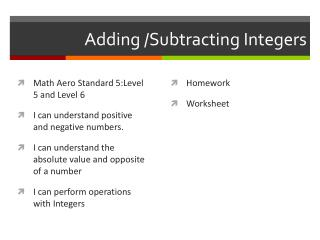DownloadDownload PresentationTélécharger la présentation- - - - - - - - - - - - - - - - - - - - - - - - - - - E N D - - - - - - - - - - - - - - - - - - - - - - - - - - -
Presentation Transcript

1. Adding /Subtracting Integers • Math Aero Standard 5:Level 5 and Level 6 • I can understand positive and negative numbers. • I can understand the absolute value and opposite of a number • I can perform operations with Integers • Homework • Worksheet

2. Warm up • Absolute Value

3. Adding integers • Think of walking along a number line . Walk forward for a positive integer and walk backward for negative integer • 12 + (-9) • -4 + (-7) • -50 + (-5)

4. Rules for adding Integers Same Sign Find the sum of absolute value Give the sum the same sign as the addends • Difference sign • Find the difference of the absolute values of the two numbers • Give the difference the sign of the addend with the greater absolute value

5. Practice • -8 + 6 • -5 + (-14) • 13 + 23 • 8 = (-16) • -3 + 14 • 5 + (-7) • 4 – (-6)

6. Subtracting Integers • Difference between minus sign and a negative number • One is an operation and the other tells you a number is negative • -7 = negative 7 • -(-6) = the opposite of negative 6 • 3-4 = 3 minus 4 • 3- (-4) = 3 minus negative 4 • -6 -7= negative 6 minus 7

7. Rule for subtracting Integers • Subtracting integers is the same as adding its opposite • 3 – (-2)= • -4 -1= • 1-5 • -6 -8=

8. Practice

9. Closure • Weekly Worksheet • Quiz